Courses
Courses for Kids
Free study material
Free LIVE classes
More

# Making and Breaking NumbersLIVE
Join Vedantu’s FREE Mastercalss

## Introduction to Making and Breaking Numbers

Hello kids! The concept of place values ​​is perhaps one of the most fundamental and essential concepts that students learn when they are new to mathematics. Only when they know the difference between the place values ​​of a number in the tens place and the one's place can they say that 100 is greater than 10 and 87 is less than 90. This lesson plan teaches students how to add a two-digit number to another two-digit number. This is done by breaking the numbers into ones, tens, and hundreds.

## Breaking Numbers

The expanded form is a way of writing a number by adding the value of its digits by breaking the number. We can use a place value chart to think about the value of the digits of a number.

Place value is the value of a digit according to its position in the number, such as ones, tens, hundreds, and so on. For example, the place value of 5 in 3458 is 5 tens, or 50. However, the place value of 5 in 5781 is expressed as 5 thousand or 5,000. It is important to understand that a digit can be the same, but its value depends on its position in the number.

Let’s stretch the valuesBreaking Numbers

In 12, the place values of the digits are given below: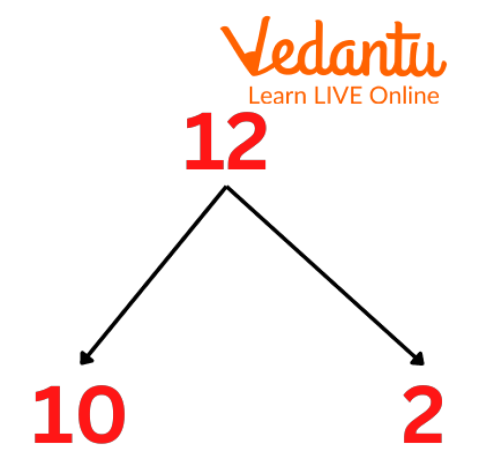Breaking Number

 1 x 10 = 10 2 x 1 = 2

In 211, the place values of the digits are given below:

 2 x 100 = 200 1 x 10 = 10 1 x 1 = 1

In 2385, the digit place values ​​are given below: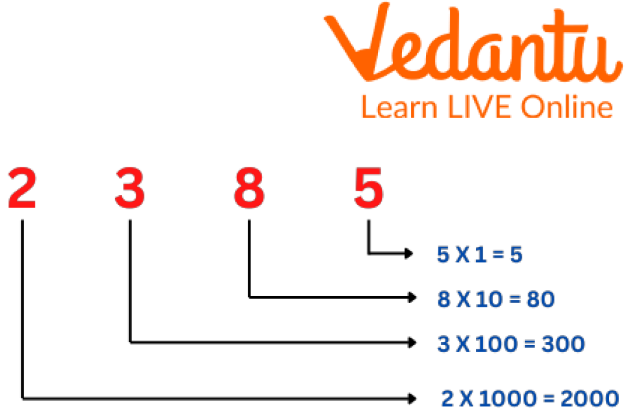Breaking Numbers

 2 x 1000 = 2000 3 x 100 = 300 8 x 10 = 80 5 x 1 5

Breaking Apart is a mental maths strategy for addition. Some students may find this method more effective than addition from left to right.

In this, we'll practice adding a two-digit number to a two-digit number by separating the numbers and then adding tens.

For example,

Example 1. We will add 14+12.

We will split the 12 into a 10 and a 2.

Now we add. First, we add 14 + 10 to make 24; then we add the remaining 2 to make 26.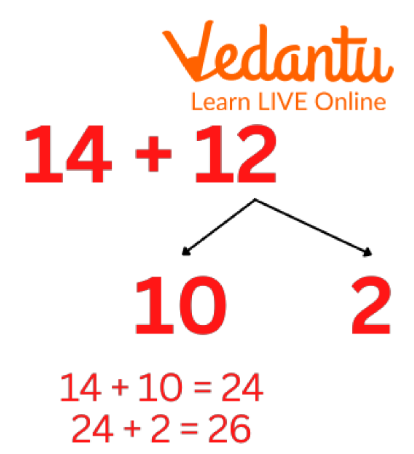Example

Example 2. 50+12

First, we Split 12 into 10 and 2.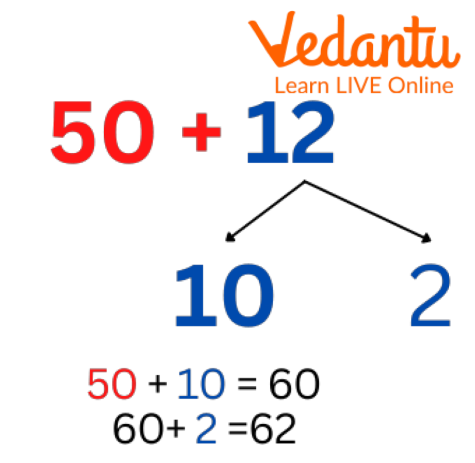Example

We add 50 + 10 to make 60,

then add the remaining 2 to make 62.

## Subtraction Using Breaking Numbers

Example 1. We will Subtract 43 - 17.

We will split the 17 into a 10 and a 7.

Then, we split 7 into 3 and 4.

Then, with the help of a line we subtract.

43 - 10 = 33

33 - 3 = 30

30 - 4 = 26

Example 2.We will Subtract 75 - 18.

We will split the 18 into a 10 and an 8.

Then, we split 8 into 5 and 3.

Then, with the help of a line we subtract.

75 - 10 = 65

65 - 5 = 60

60 - 3 = 57

Example 3. We will Subtract 75 - 12.

We will split the 12 into a 10 and a 2.

Then, with the help of a line we subtract.

75 - 10 = 65

65 - 2 = 63

## Solved Examples

1. Breaking numbers:

 14 10 +4 56 24 35 92 81

Ans: After breaking numbers:

 14 10 +4 56 50 + 6 24 20 + 4 35 30 + 5 92 90 + 2 81 80 + 1

2. Adding by Breaking Apart Tens and Ones.

a. Break apart the numbers into tens and ones to add.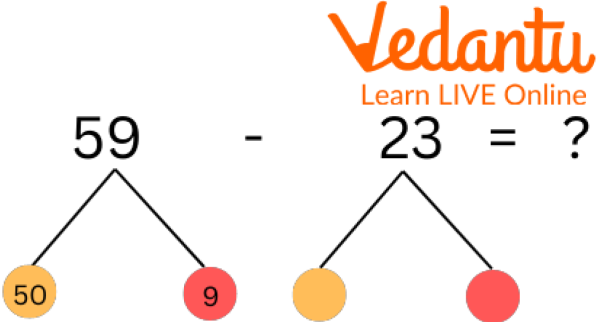Solved Example

Ans: 36

b.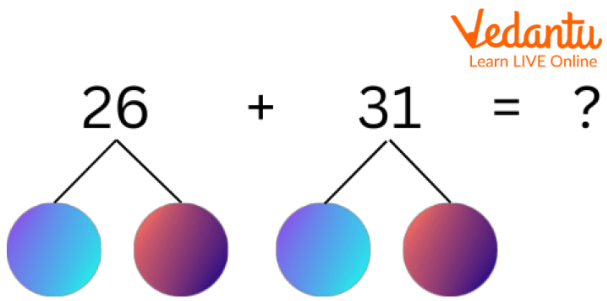Solved Example

Ans: 57

## Practice Questions

Q1. Break the given numbers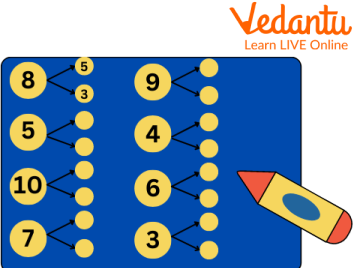Practice Question

Ans: 9 = 4 + 5

5 = 2 + 3

4 = 3 + 1

10 = 6 + 4

6 = 4 + 2

7 = 6 + 1

3 = 2 + 1

## Summary

In this article, we learned about place values, its importance and how we use them to make or break numbers. We also learned to separate tens and ones by breaking the tens digit and further using them to solve questions in addition, subtraction etc. For example, 56 = 50 + 6. Along with that, we learned more about it by solving numerous questions to grasp breaking numbers.

Last updated date: 23rd Sep 2023
Total views: 83.7k
Views today: 1.83k

## FAQs on Making and Breaking Numbers

1. What is the term to separate a large number?

The factorisation factorises a number quickly into smaller numbers or factors of the number. A factor is a number that divides the given number without any remainder.

2. What are the 4 basic arithmetic operations?

The four basic mathematical operations are addition, subtraction, multiplication and division.

3. Who is the father of maths?

A Greek mathematician Archimedes is known as the Father of Mathematics. He is considered the Father of Mathematics for his significant contribution to the development of mathematics.